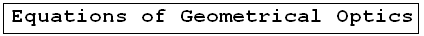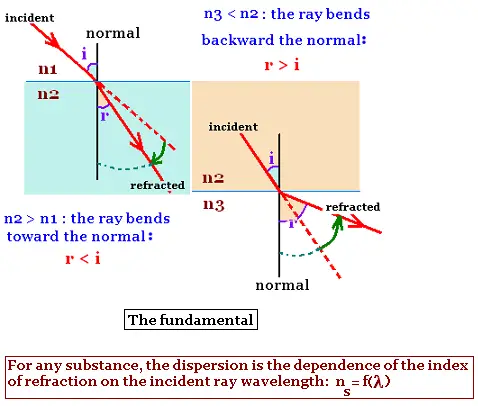Geometrical Optics: Geometrical Optics & Physics Optics. a Huygens Principle: Wavefronts. a Reflection: The first law of Geometrical Optics. a Refraction: The second law of Geometrical Optics: Snell's law. a Critical angle: Internal total reflection. a images formed by reflection Spherical concave mirrors. Mirror equation. a images formed by refraction Spherical convex mirrors .. a Fermat's principle: Fermat's principle for reflection and refraction.. a lenses: Thin lenses and Lens-makers' equation .. a prism: Prism, colors minimum deviation .. a Human eye: near point, far point, nearsightedness, farsightedness.. a Microscope: Microscope: simple, compound, and magnification.. a Telescope: Telescope: magnification.. a Resolution: optical microscope, electron microscope .. a dispersion: dispersion, more about the refractive index, and colors of a prism .. a parallel sheet: deviation by a parallel sheet .. a thin films : thin films and Newton's rings.. a Newton's rings: thin films and Newton's rings.. a Descartes rainbow: Primary and secondary rainbows .. a Some applications More fun with Optics .. a Optics calculator: Optics calculator: all the related calculations .. a home The fundamental, and just this .. a scientificSentence a __________________ a

Geometical Optics@import url(http://www.google.com/cse/api/branding.css);Custom Search © 2009. The scientificsentence . All rights reserved.